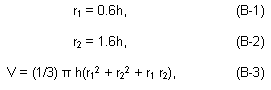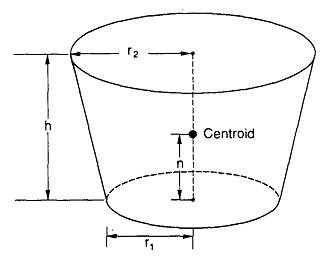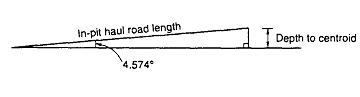APPENDIX B. OPEN PIT GEOMETRY

All base case calculations use a SR of 1:1, and a ton­nage to volume relationship of 2.225 st/BCY (1.357 st/ LCY).

The idealized pit geometry is a frustum of a right circular cone, as illustrated in figure B-1. The following relationships apply for this assumed pit shape:distance from the surface of the base whose radius is r1 to the centroid is:

Figure B-1. Assumed pit shape for open pit mine models (frustum of a right circular cone).where n = distance from base of pit to centroid,

h = height of cone (or depth of pit),

r1 = radius of lower base,

r2 = radius of upper base,

V = volume of cone (pit).

Depth to centroid (depth of pit minus distance to centroid from the base) is calculated using the following:

depth to centroid = h - n. (B-5)

Haul distance from centroid to edge of pit, assuming an in-pit haul road grade of 8% (4.574°) (fig. B-2):

in-pit haul road length = depth to centroid/sin 4.574°. (B-6)

Figure B-2.-,Assumed slope of in-pit haul roads for open pit mine models.